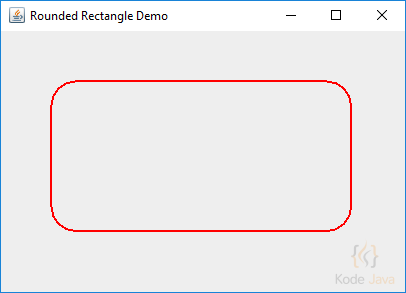# How do I draw a round rectangle in Java 2D?

The `RoundRectangle2D` class defines a rectangle with rounded corners defined by a location `(x,y)`, dimension `(w x h)`, and the width and height of an arc with which to round the corners.

The `RoundRectangle2D.Double` class constructs a `RoundRectangle2D` from the specified values in double, including the location, width and the arch of the round rectangle.

``````package org.kodejava.example.geom;

import javax.swing.*;
import java.awt.*;
import java.awt.geom.RoundRectangle2D;

public class DrawRoundRectangle extends JComponent {
@Override
public void paint(Graphics g) {
Graphics2D g2 = (Graphics2D) g;

g2.setPaint(Color.RED);
g2.setStroke(new BasicStroke(2.0f));

double x = 50;
double y = 50;
double w = x + 250;
double h = y + 100;
g2.draw(new RoundRectangle2D.Double(x, y, w, h, 50, 50));
}

public static void main(String[] args) {
JFrame frame = new JFrame("Rounded Rectangle Demo");
frame.setDefaultCloseOperation(WindowConstants.EXIT_ON_CLOSE);
frame.getContentPane().add(new DrawRoundRectangle(), BorderLayout.CENTER);
frame.pack();
frame.setSize(420, 300);
frame.setVisible(true);
}
}
``````

Here is the result you’ll get when you run the snippet:This site uses Akismet to reduce spam. Learn how your comment data is processed.